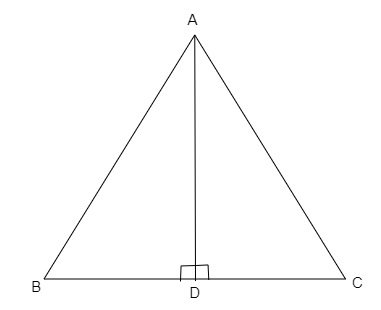# In an equilateral $\triangle ABC$, $AD \perp BC$, prove that $AD^2=3BD^2$.

Given:

In an equilateral $\triangle ABC$, $AD \perp BC$.
To do:

We have to  prove that $AD^2=3BD^2$.

Solution:In $\triangle ADB$ and $\triangle ACD$,

$\angle ADB=\angle ADC=90^o$

$AB=AC$

$AD=AD$     (Common side)

Therefore,

$\triangle ADB \cong\ \triangle ACD$    (By RHS congruence)

This implies,

$BD=DC=\frac{BC}{2}$     (CPCT)

In $\triangle ADB$,

$AB^2=AD^2+BD^2$    (By using Pythagoras theorem)

$BC^2=AD^2+BD^2$    (Since $AB=AC$)

$(2BD)^2=AD^2+BD^2$

$4BD^2=AD^2+BD^2$

$(4-1)BD^2=AD^2$

$AD^2=3BD^2$

Hence proved.

Updated on: 10-Oct-2022

45 Views